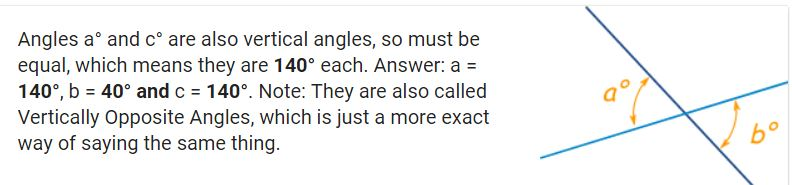# ANGLES:

## Complementary, Supplementary, Vertical

### MEMORY TRICK:

"C" of Complementary stands for "Corner" (90 degrees in a right angle),
"S" of Supplementary stands for "Straight" (180 degrees is a straight line)# Transversal VIDEO Lessons:

1-- Video #1057- Find-measurement of an angle in a triangle using the other two angles

2-- Video #1229 - Find measurement of an exterior angle

3-- Video #1248 - Find the side length of a triangle using angle-angle criterion

4-- Video #1236 - Find the measurements of corresponding angles

5-- Video #1237 - Find measurements of vertical and adjacent-angles

6-- Video #1241- Find measurements of alternate-interior and alternate exterior-angles

7-- Video #382 Proof of the Pythagorean Theorem
Notes: x + y + 90 = 180 degrees (sides of triangle)
3 angles form a line = 180 degrees ... (stop video at 8:49 min)
8-- Video ADVANCED LESSON: Converse of the Pythageom Theorem
(triples to memorize)
 INTERACTIVE PRACTICE: Identify Angles: Complementary, supplementary, vertical, adjacent and congruent INTERACTIVE PRACTICE: Find measures of complementary, supplementary, vertical, and adjacent angles INTERACTIVE PRACTICE: Parallel Lines with a Transversal (and vertical angles)

# VOCABULARY: LINES

 Transversal is a line that crosses two or more lines. Transversal Parallel Lines: Two lines are parallel if they lie in the same plane and they do not intersect. A straight line measures 180 degrees. Parallel Lines

# VOCABULARY: ANGLES

 Angle is a region between two rays or the amount of rotation about a fixed point. An angle is represented by two line segments or two rays that have a common endpoint (point of rotation). Angle Complementary angles are two angles whose sum is 90 degrees. Complementary angles Supplementary Angles = 180 degrees. Supplementary Angle Congruent angles have the same measure. Congruent Angle Adjacent angels share a vertex and side (like Neighbor's share a fence). Adjacent angles Vertical angles are congruent angles formed opposite each other when two lines intersect. Vertical Lines: a pair of non-adjacent angles formed by the interseftion of two straight lines. Verical Lines animated view View notes: Vertical Lines Vertical Angles VERTICAL ANGLES in transversal cut parallel line (CORRESPONDING ANGLES) Interior angles of a triangle = 180 degrees. Interior angles of quadrilaterals = 360 degrees. Interior angle Exterior Angle: An exterior angle of a polygon is formed when you extend a side of a polygon. Also, when a third line (a transversal) crosses two other lines, the angles formed outside the other two lines are called exterior angles. (see alternate exterior angles) Exterior Angle Alternate Interior Angles: Alternate interior angles are pairs of angles formed when a third line (a transversal) crosses two other lines. These angles are on opposite sides of the transversal and are in between the other two lines. Alternate Interior Angles Alternate exterior angles are pairs of angles formed when a third line (a transversal) crosses two other lines. These angles are on opposite sides of the transversal and are outside the other two lines. Alternate Exterior Angles

# RELATED TERMS

 Corresponding Angles: Angles that have the same relative positions in geometric figures. Vertical Angles: Two nonadjacent angles formed by intersecting lines or segments. Also called opposite angles. Ray: A ray begins at a point and goes on forever in one direction. Vertex of an Angle: The common endpoint of the two rays that serve as the sides of an angle. Corresponding Angles: Angles that have the same relative positions in geometric figures. Plane: One of the basic undefined terms of geometry. A plane goes on forever in all directions (in two-dimensions) and is "flat" (i.e., it has no thickness). Line: One of the basic undefined terms of geometry. A line has no thickness but its length goes on forever in two directions. Intersecting Lines: Two lines that cross each other. Lines intersect at one point unless the lines fall directly on top of each other (in which case they are essentially the same line and are sometimes called coincidental). Perpendicular Lines: Two lines are perpendicular if they intersect at a right angle.

 All rights reserved. [ page created 2012 by Janet McGinn ]